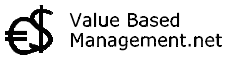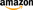## Calculation of NPVThe Net Present Value (NPV) of an investment (project) is the difference between the sum of the discounted cash flows which are expected from the investment and the amount which is initially invested. It is a traditional valuation method (often for a project) used in the Discounted Cash Flow measurement methodology, whereby the following steps are undertaken:   Calculation of expected free cash flows (often per per year) that result out of the investment Subtract /discount for the cost of capital (an interest rate to adjust for time and risk) The intermediate result is called: Present Value (PV). Subtract the initial investments The end result is called: Net Present Value (NPV).   So NPV is an amount that expresses how much value an investment will result in. This is done by measuring all cash flows over time back towards the current point in present time.   If the NPV method results in a positive amount, the project should be undertaken.   Although NPV measurement is widely used for making investment decisions, a disadvantage of NPV is that it does not account for flexibility / uncertainty after the project decision. See Real Options for more information regarding dealing with this in valuation.   Book: S. David Young, Stephen F. O'Byrne - EVA and Value-Based Management: A Practical Guide to Implementation -Book: Aswath Damodaran - Investment Valuation: Tools and Techniques for Determining the Value of Any Asset -Book: James R. Hitchner - Financial Valuation: Applications and Models -Compare with Net Present Value: Internal Rate of Return  |  Payback Period  |  Cost-Benefit Analysis